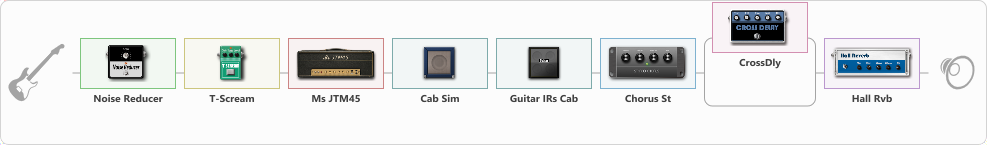# Crunch blues strat one

Discussion in 'ToneLib-GFX presets' started by truss van halen, Dec 14, 2020.

1. ### truss van halenWell-Known Member

Crunch blues strat one

Preset name: Crunch blues strat

Effects chain:Effect: "Noise Reducer" (Dynamics / Filter), active - "yes"
{
"Sens" = 100
"Mode" = Hard
}

Effect: "T-Scream" (Overdrive / Distortion), active - "yes"
{
"Drive" = 0
"Tone" = 40
"Level" = 79
}

Effect: "Ms JTM45" (Amp simulators), active - "yes"
{
"Gain" = 74
"Bass" = 56
"Middle" = 63
"Treble" = 59
"Presence" = 50
"Master" = 64
"Level (dB)" = 6
}

Effect: "Cab Sim" (Cabinets), active - "yes"
{
"Model" = 2x12" Silver Bell
"Level (dB)" = 0
}

Effect: "Guitar IRs Cab" (Cabinets), active - "yes"
{
"Model" = Fender Deluxe Rev (1x12")
"Mic Position" = Middle
"Mic Distance" = Near
"Low Cut (Hz)" = 60
"Hi Cut (kHz)" = 20.0
"Mix" = 100
"Level (dB)" = 0
}

Effect: "Chorus St" (Modulation / Sfx), active - "yes"
{
"Speed" = 3.2
"Depth" = 51
"Center" = 6.4
"Mix" = 100
}

Effect: "Splitter" (Dynamics / Filter), active - "yes"
{
"A-Bypass" = Off
"A-Pan" = 0
"A-Level" = 55
"B-Bypass" = Off
"B-Pan" = 0
"B-Level" = 55

'A' branch:
{

Effect: "CrossDly" (Delay), active - "yes"
{
"Time" = 756
"Feedback" = 53
"Tone" = 80
"Sens" = 38
"Mix" = 43
}
}
'B' branch:
{
}
}

Effect: "Hall Rvb" (Reverberation), active - "yes"
{
"Time" = 9.4
"PreDelay" = 61
"LoDamp" = 49
"HiDamp" = 17
"Mix" = 44
}

Note: You will need to download and install the ToneLib-GFX software to use the preset.

#### Attached Files:

• ###### Crunch_blues_strat_one.tlgfx
File size:
869 bytes
Views:
3,088
2. Hi,

how can i use it for g1 four pedal? i can't add the file to the tonelib software.